# RD Sharma Solutions for Class 11 Chapter 19 - Arithmetic Progressions Exercise 19.4

In this section, we shall discuss problems based on the sum to n terms of an A.P. This exercise can be used as a model of reference by the students to improve their conceptual knowledge and understand the different ways used to solve the problems. Here, the exercise wise problems are solved and explained in simple language by using various methods and steps. Students mainly require lots of practice to analyse the types of problems that would appear in the exam. By regular practice, students can improve their logical approach to solve the problems. RD Sharma Class 11 Maths Solutions pdf links are provided below, where students can easily download and start practising offline.

## Download the pdf of RD Sharma Solutions for Class 11 Maths Exercise 19.4 Chapter 19 – Arithmetic Progressions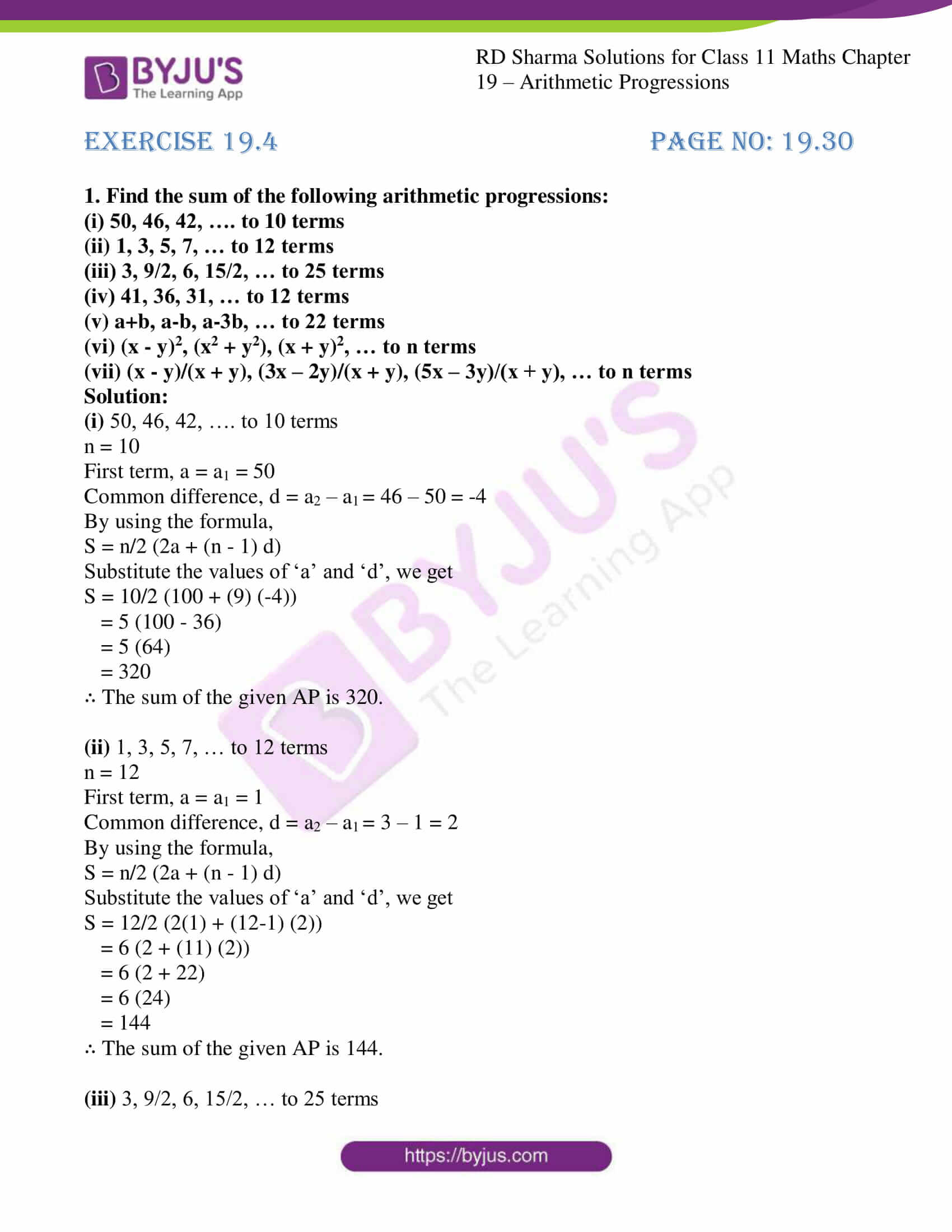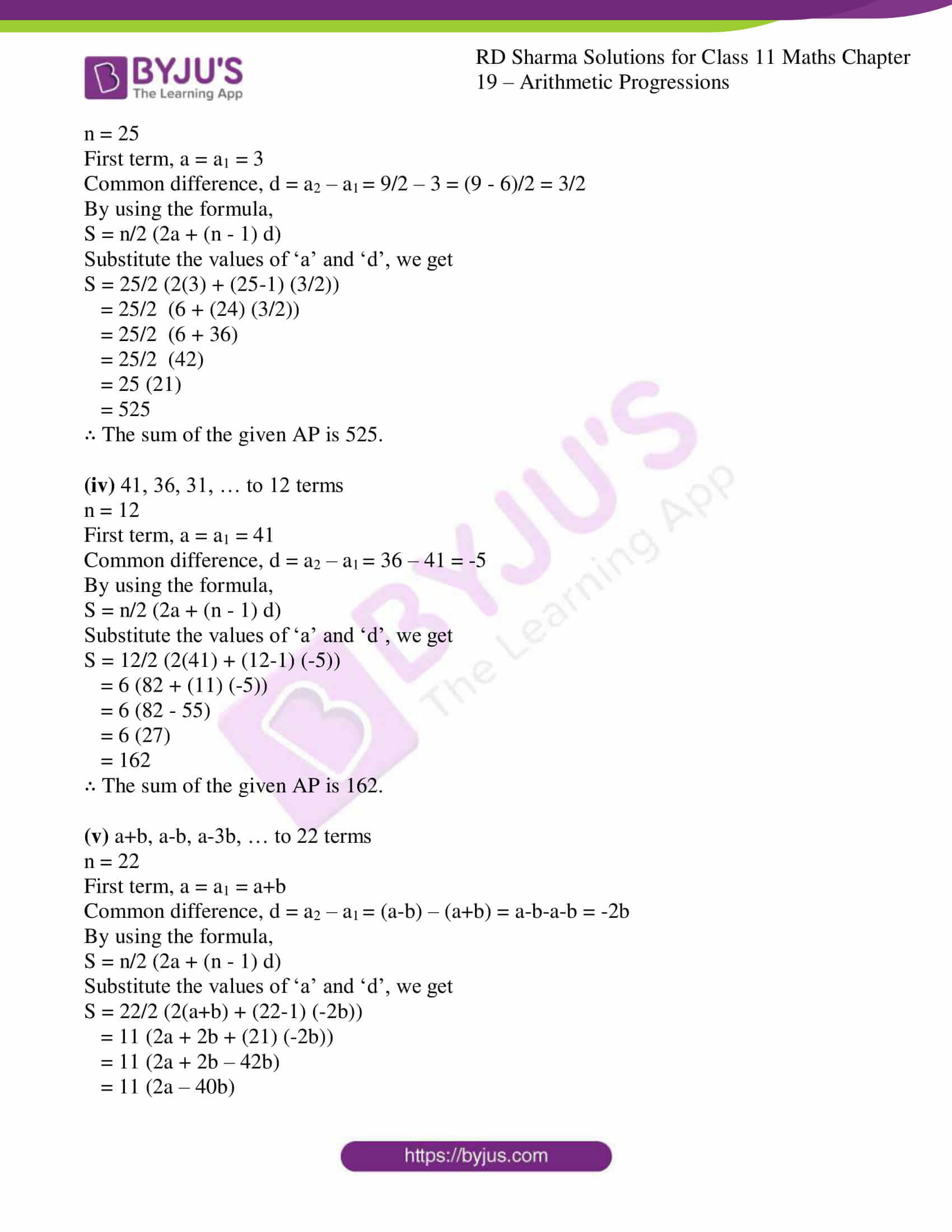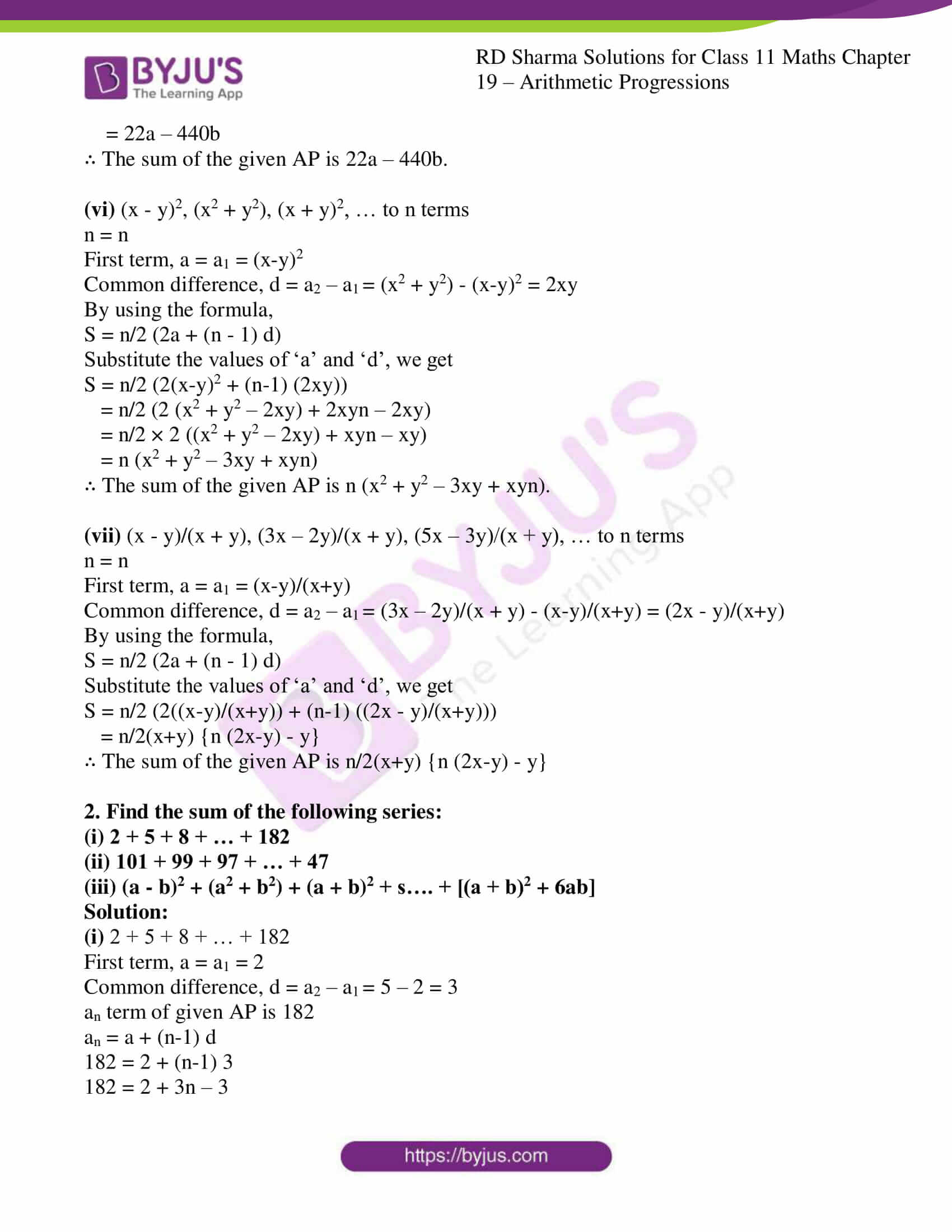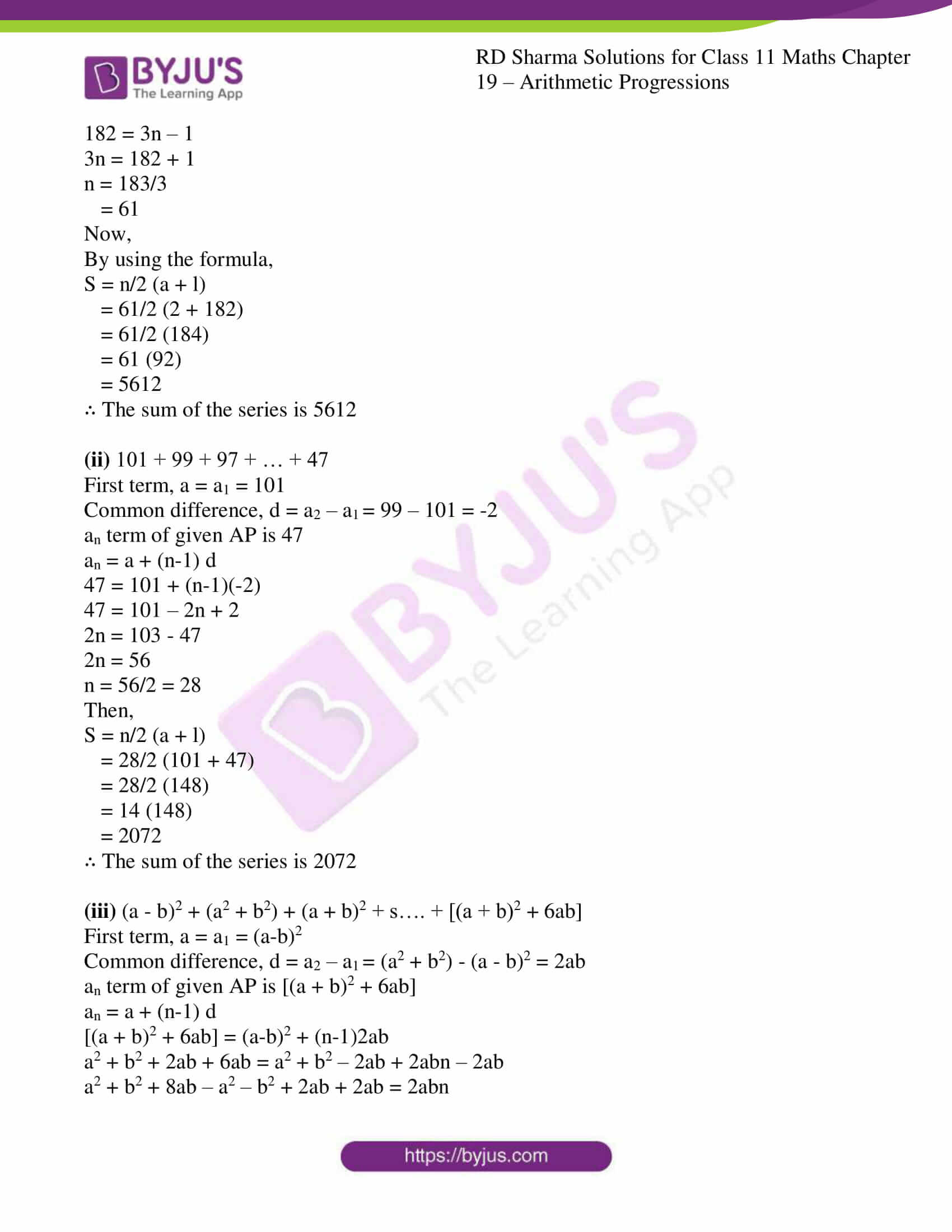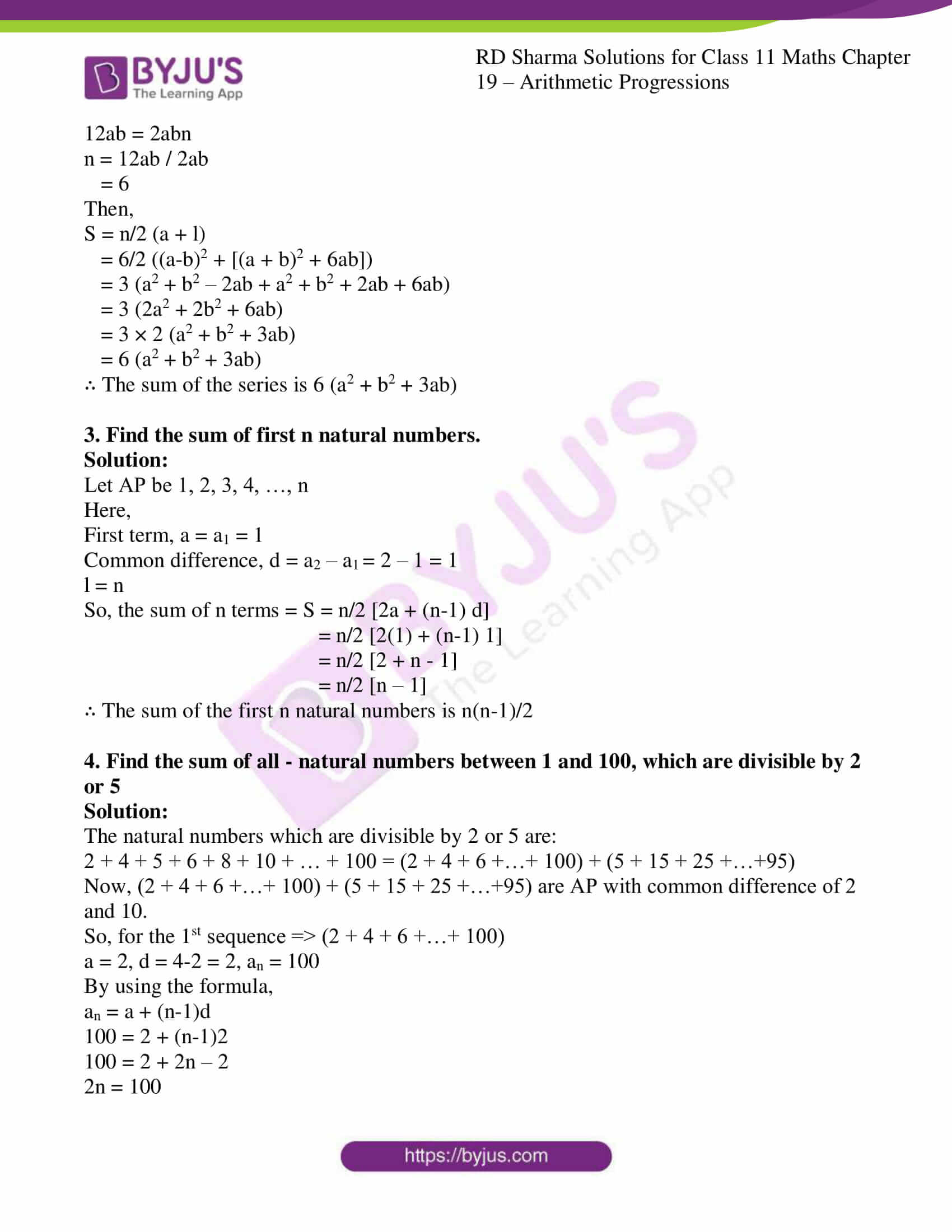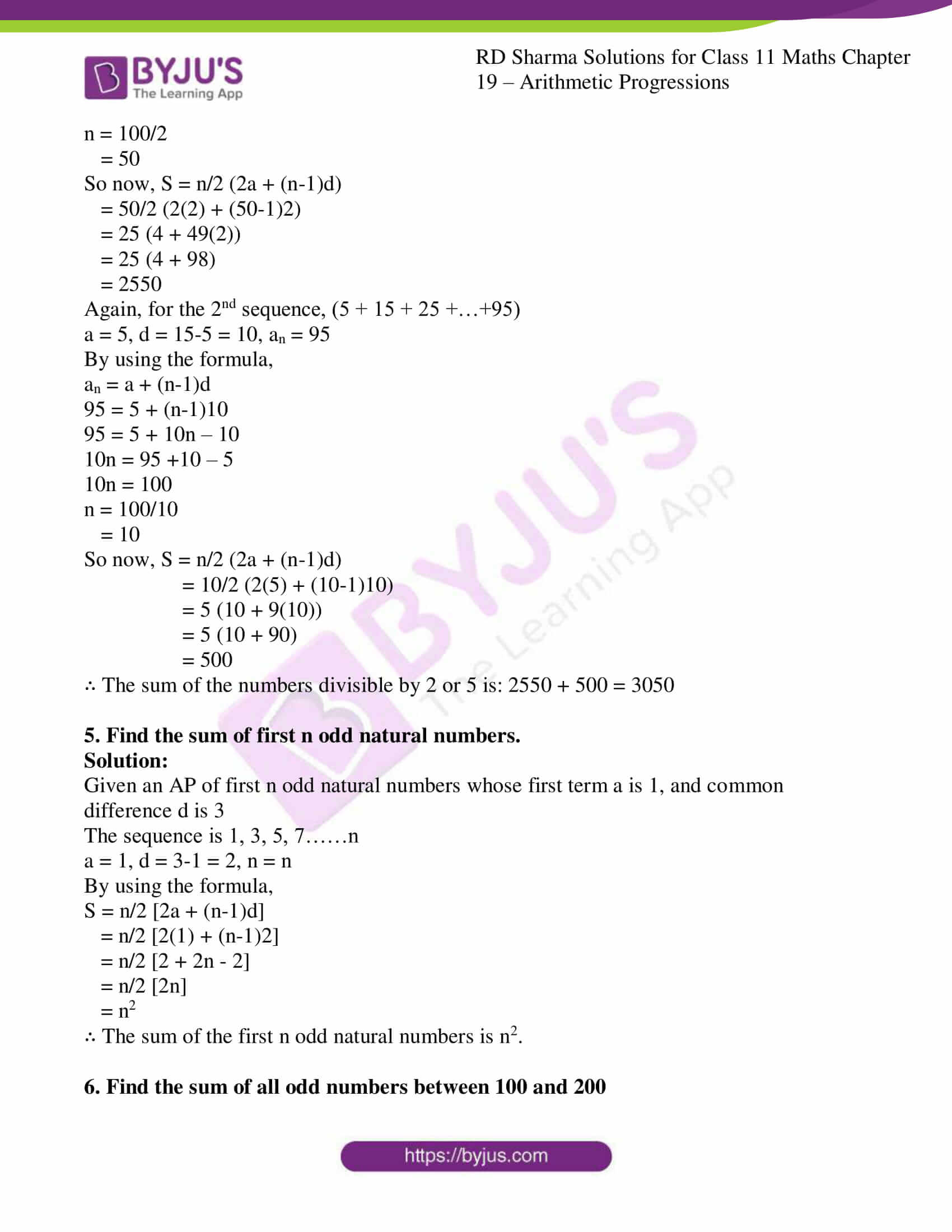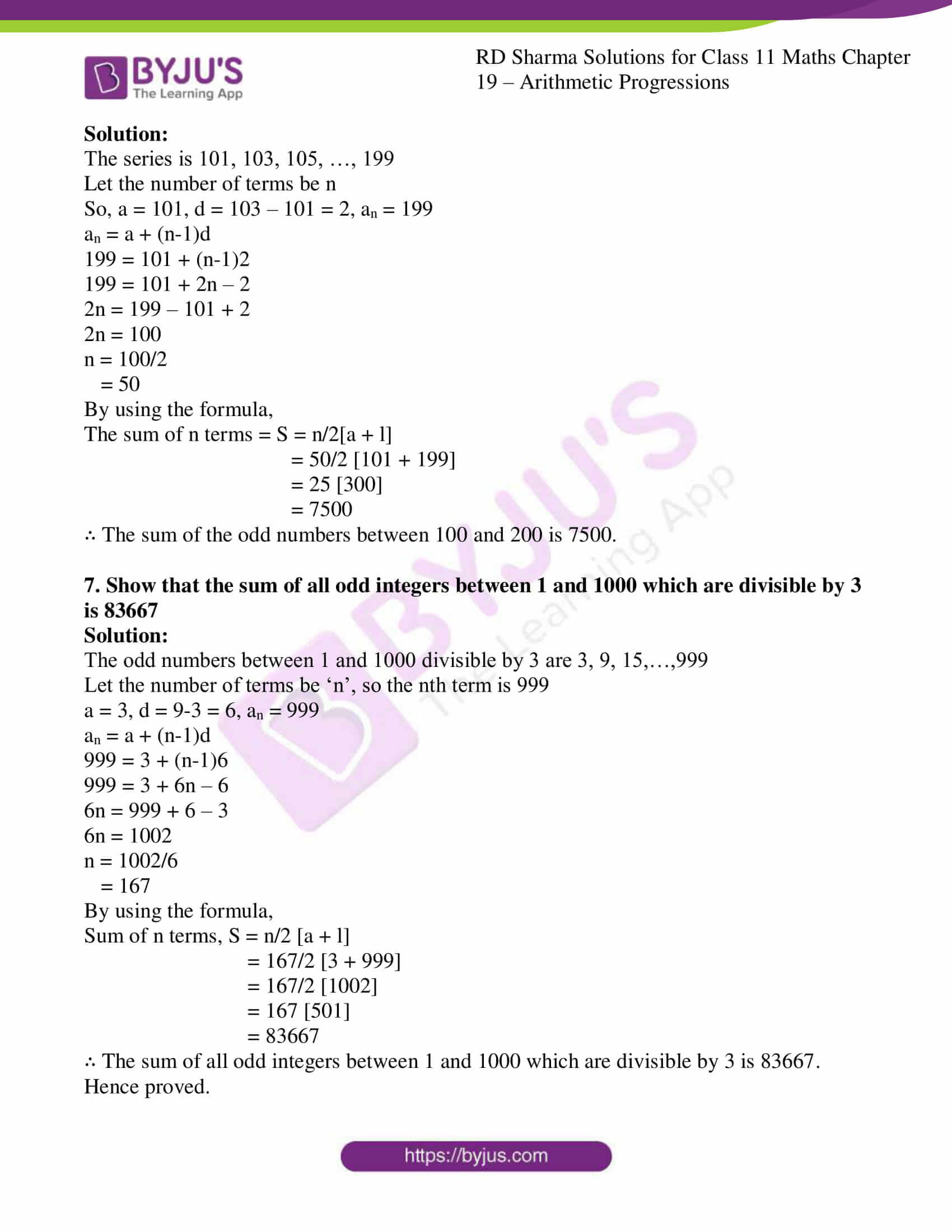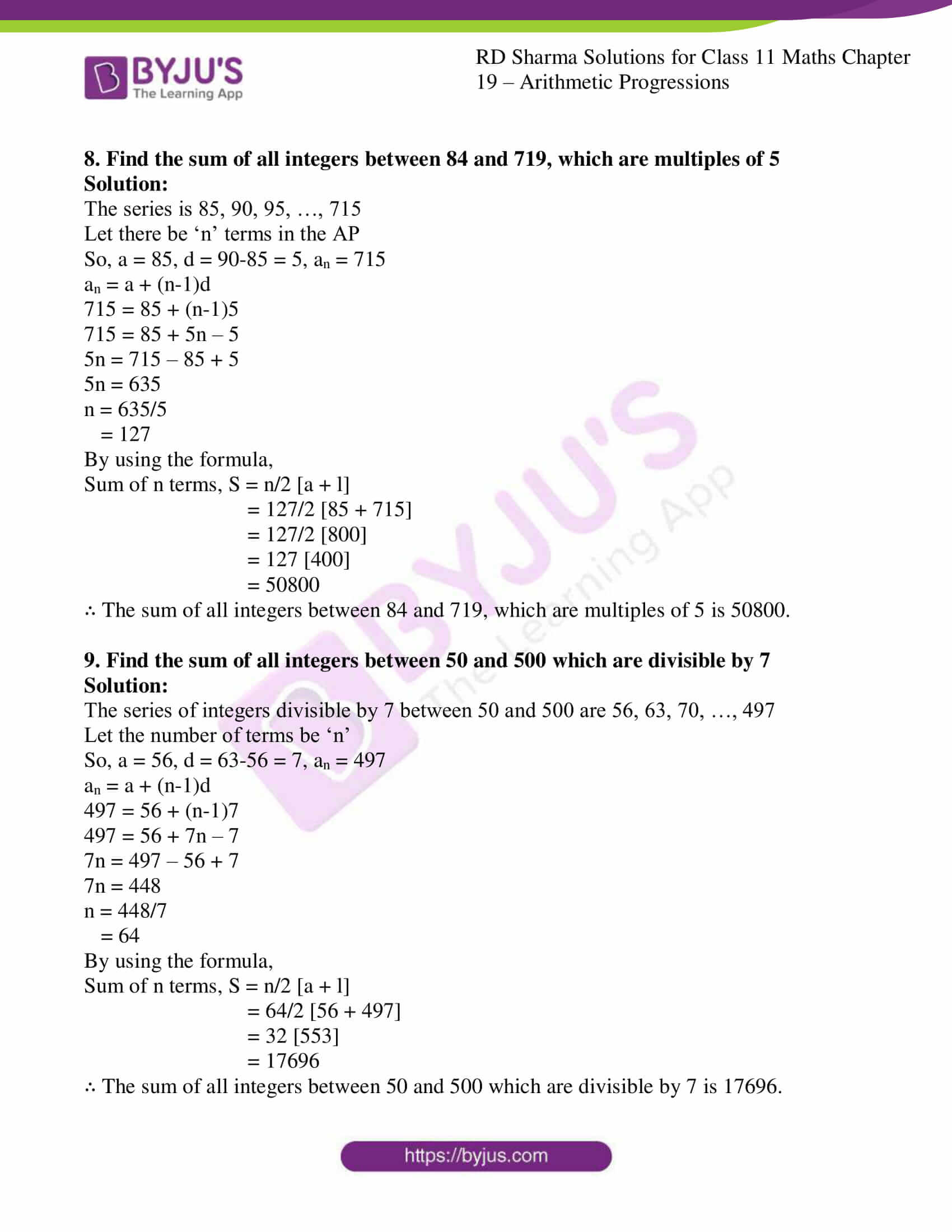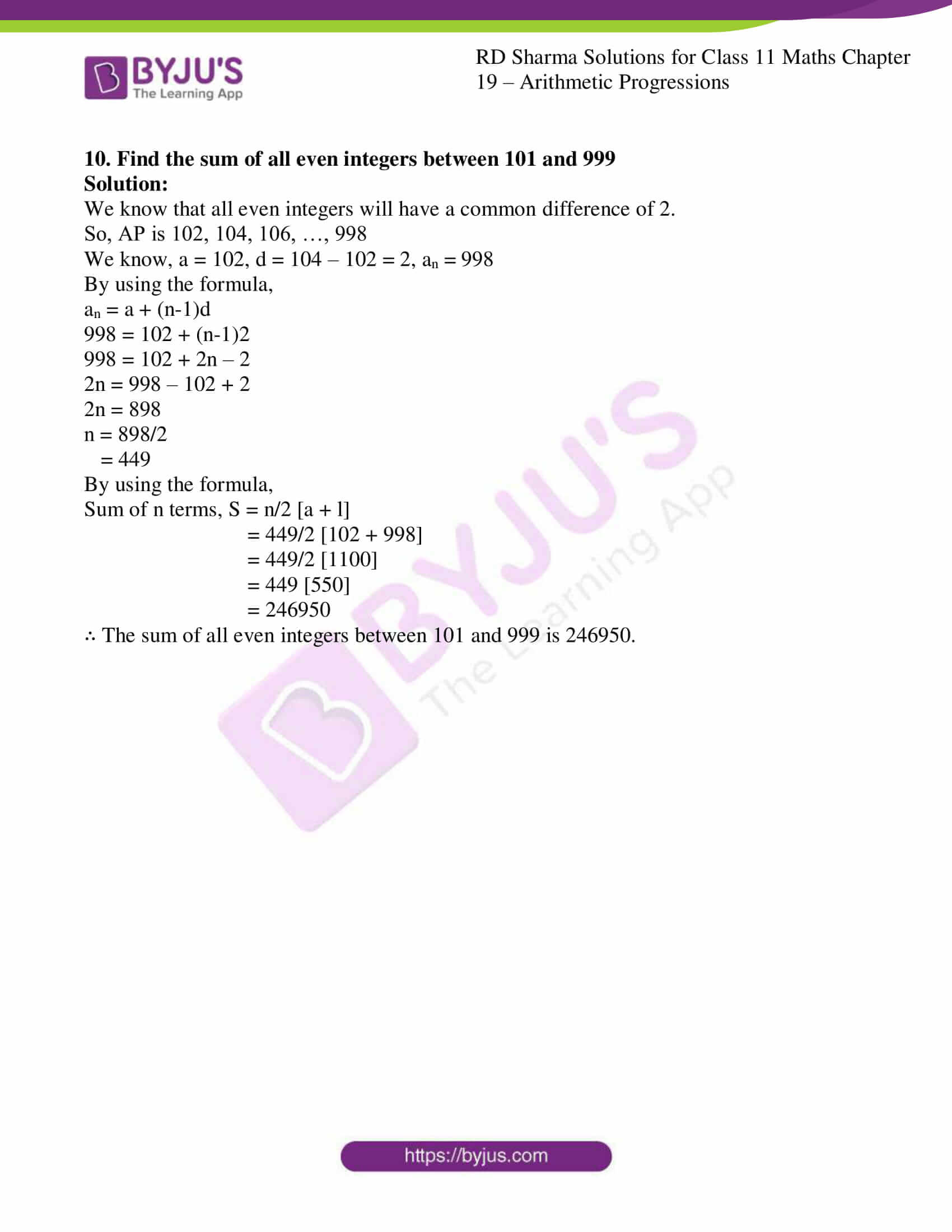### Also, access other exercises of RD Sharma Solutions for Class 11 Maths Chapter 19 – Arithmetic Progressions

Exercise 19.1 Solutions

Exercise 19.2 Solutions

Exercise 19.3 Solutions

Exercise 19.5 Solutions

Exercise 19.6 Solutions

Exercise 19.7 Solutions

### Access answers to RD Sharma Solutions for Class 11 Maths Exercise 19.4 Chapter 19 – Arithmetic Progressions

1. Find the sum of the following arithmetic progressions:
(i) 50, 46, 42, …. to 10 terms

(ii) 1, 3, 5, 7, … to 12 terms

(iii) 3, 9/2, 6, 15/2, … to 25 terms

(iv) 41, 36, 31, … to 12 terms

(v) a+b, a-b, a-3b, … to 22 terms

(vi) (x – y)2, (x2 + y2), (x + y)2, … to n terms

(vii) (x – y)/(x + y), (3x – 2y)/(x + y), (5x – 3y)/(x + y), … to n terms

Solution:

(i) 50, 46, 42, …. to 10 terms

n = 10

First term, a = a1 = 50

Common difference, d = a2 – a1 = 46 – 50 = -4

By using the formula,

S = n/2 (2a + (n – 1) d)

Substitute the values of ‘a’ and ‘d’, we get

S = 10/2 (100 + (9) (-4))

= 5 (100 – 36)

= 5 (64)

= 320

∴ The sum of the given AP is 320.

(ii) 1, 3, 5, 7, … to 12 terms

n = 12

First term, a = a1 = 1

Common difference, d = a2 – a1 = 3 – 1 = 2

By using the formula,

S = n/2 (2a + (n – 1) d)

Substitute the values of ‘a’ and ‘d’, we get

S = 12/2 (2(1) + (12-1) (2))

= 6 (2 + (11) (2))

= 6 (2 + 22)

= 6 (24)

= 144

∴ The sum of the given AP is 144.

(iii) 3, 9/2, 6, 15/2, … to 25 terms

n = 25

First term, a = a1 = 3

Common difference, d = a2 – a1 = 9/2 – 3 = (9 – 6)/2 = 3/2

By using the formula,

S = n/2 (2a + (n – 1) d)

Substitute the values of ‘a’ and ‘d’, we get

S = 25/2 (2(3) + (25-1) (3/2))

= 25/2 (6 + (24) (3/2))

= 25/2 (6 + 36)

= 25/2 (42)

= 25 (21)

= 525

∴ The sum of the given AP is 525.

(iv) 41, 36, 31, … to 12 terms

n = 12

First term, a = a1 = 41

Common difference, d = a2 – a1 = 36 – 41 = -5

By using the formula,

S = n/2 (2a + (n – 1) d)

Substitute the values of ‘a’ and ‘d’, we get

S = 12/2 (2(41) + (12-1) (-5))

= 6 (82 + (11) (-5))

= 6 (82 – 55)

= 6 (27)

= 162

∴ The sum of the given AP is 162.

(v) a+b, a-b, a-3b, … to 22 terms

n = 22

First term, a = a1 = a+b

Common difference, d = a2 – a1 = (a-b) – (a+b) = a-b-a-b = -2b

By using the formula,

S = n/2 (2a + (n – 1) d)

Substitute the values of ‘a’ and ‘d’, we get

S = 22/2 (2(a+b) + (22-1) (-2b))

= 11 (2a + 2b + (21) (-2b))

= 11 (2a + 2b – 42b)

= 11 (2a – 40b)

= 22a – 440b

∴ The sum of the given AP is 22a – 440b.

(vi) (x – y)2, (x2 + y2), (x + y)2, … to n terms

n = n

First term, a = a1 = (x-y)2

Common difference, d = a2 – a1 = (x2 + y2) – (x-y)2 = 2xy

By using the formula,

S = n/2 (2a + (n – 1) d)

Substitute the values of ‘a’ and ‘d’, we get

S = n/2 (2(x-y)2 + (n-1) (2xy))

= n/2 (2 (x2 + y2 – 2xy) + 2xyn – 2xy)

= n/2 × 2 ((x2 + y2 – 2xy) + xyn – xy)

= n (x2 + y2 – 3xy + xyn)

∴ The sum of the given AP is n (x2 + y2 – 3xy + xyn).

(vii) (x – y)/(x + y), (3x – 2y)/(x + y), (5x – 3y)/(x + y), … to n terms

n = n

First term, a = a1 = (x-y)/(x+y)

Common difference, d = a2 – a1 = (3x – 2y)/(x + y) – (x-y)/(x+y) = (2x – y)/(x+y)

By using the formula,

S = n/2 (2a + (n – 1) d)

Substitute the values of ‘a’ and ‘d’, we get

S = n/2 (2((x-y)/(x+y)) + (n-1) ((2x – y)/(x+y)))

= n/2(x+y) {n (2x-y) – y}

∴ The sum of the given AP is n/2(x+y) {n (2x-y) – y}

2. Find the sum of the following series:
(i) 2 + 5 + 8 + … + 182

(ii) 101 + 99 + 97 + … + 47

(iii) (a – b)2 + (a2 + b2) + (a + b)2 + s…. + [(a + b)2 + 6ab]

Solution:

(i) 2 + 5 + 8 + … + 182

First term, a = a1 = 2

Common difference, d = a2 – a1 = 5 – 2 = 3

an term of given AP is 182

an = a + (n-1) d

182 = 2 + (n-1) 3

182 = 2 + 3n – 3

182 = 3n – 1

3n = 182 + 1

n = 183/3

= 61

Now,

By using the formula,

S = n/2 (a + l)

= 61/2 (2 + 182)

= 61/2 (184)

= 61 (92)

= 5612

∴ The sum of the series is 5612

(ii) 101 + 99 + 97 + … + 47

First term, a = a1 = 101

Common difference, d = a2 – a1 = 99 – 101 = -2

an term of given AP is 47

an = a + (n-1) d

47 = 101 + (n-1)(-2)

47 = 101 – 2n + 2

2n = 103 – 47

2n = 56

n = 56/2 = 28

Then,

S = n/2 (a + l)

= 28/2 (101 + 47)

= 28/2 (148)

= 14 (148)

= 2072

∴ The sum of the series is 2072

(iii) (a – b)2 + (a2 + b2) + (a + b)2 + s…. + [(a + b)2 + 6ab]

First term, a = a1 = (a-b)2

Common difference, d = a2 – a1 = (a2 + b2) – (a – b)2 = 2ab

an term of given AP is [(a + b)2 + 6ab]

an = a + (n-1) d

[(a + b)2 + 6ab] = (a-b)2 + (n-1)2ab

a2 + b2 + 2ab + 6ab = a2 + b2 – 2ab + 2abn – 2ab

a2 + b2 + 8ab – a2 – b2 + 2ab + 2ab = 2abn

12ab = 2abn

n = 12ab / 2ab

= 6

Then,

S = n/2 (a + l)

= 6/2 ((a-b)2 + [(a + b)2 + 6ab])

= 3 (a2 + b2 – 2ab + a2 + b2 + 2ab + 6ab)

= 3 (2a2 + 2b2 + 6ab)

= 3 × 2 (a2 + b2 + 3ab)

= 6 (a2 + b2 + 3ab)

∴ The sum of the series is 6 (a2 + b2 + 3ab)

3. Find the sum of first n natural numbers.

Solution:

Let AP be 1, 2, 3, 4, …, n

Here,

First term, a = a1 = 1

Common difference, d = a2 – a1 = 2 – 1 = 1

l = n

So, the sum of n terms = S = n/2 [2a + (n-1) d]

= n/2 [2(1) + (n-1) 1]

= n/2 [2 + n – 1]

= n/2 [n – 1]

∴ The sum of the first n natural numbers is n(n-1)/2

4. Find the sum of all – natural numbers between 1 and 100, which are divisible by 2 or 5

Solution:

The natural numbers which are divisible by 2 or 5 are:

2 + 4 + 5 + 6 + 8 + 10 + … + 100 = (2 + 4 + 6 +…+ 100) + (5 + 15 + 25 +…+95)

Now, (2 + 4 + 6 +…+ 100) + (5 + 15 + 25 +…+95) are AP with common difference of 2 and 10.

So, for the 1st sequence => (2 + 4 + 6 +…+ 100)

a = 2, d = 4-2 = 2, an = 100

By using the formula,

an = a + (n-1)d

100 = 2 + (n-1)2

100 = 2 + 2n – 2

2n = 100

n = 100/2

= 50

So now, S = n/2 (2a + (n-1)d)

= 50/2 (2(2) + (50-1)2)

= 25 (4 + 49(2))

= 25 (4 + 98)

= 2550

Again, for the 2nd sequence, (5 + 15 + 25 +…+95)

a = 5, d = 15-5 = 10, an = 95

By using the formula,

an = a + (n-1)d

95 = 5 + (n-1)10

95 = 5 + 10n – 10

10n = 95 +10 – 5

10n = 100

n = 100/10

= 10

So now, S = n/2 (2a + (n-1)d)

= 10/2 (2(5) + (10-1)10)

= 5 (10 + 9(10))

= 5 (10 + 90)

= 500

∴ The sum of the numbers divisible by 2 or 5 is: 2550 + 500 = 3050

5. Find the sum of first n odd natural numbers.

Solution:

Given an AP of first n odd natural numbers whose first term a is 1, and common difference d is 3

The sequence is 1, 3, 5, 7……n

a = 1, d = 3-1 = 2, n = n

By using the formula,

S = n/2 [2a + (n-1)d]

= n/2 [2(1) + (n-1)2]

= n/2 [2 + 2n – 2]

= n/2 [2n]

= n2

∴ The sum of the first n odd natural numbers is n2.

6. Find the sum of all odd numbers between 100 and 200

Solution:

The series is 101, 103, 105, …, 199

Let the number of terms be n

So, a = 101, d = 103 – 101 = 2, an = 199

an = a + (n-1)d

199 = 101 + (n-1)2

199 = 101 + 2n – 2

2n = 199 – 101 + 2

2n = 100

n = 100/2

= 50

By using the formula,

The sum of n terms = S = n/2[a + l]

= 50/2 [101 + 199]

= 25 

= 7500

∴ The sum of the odd numbers between 100 and 200 is 7500.

7. Show that the sum of all odd integers between 1 and 1000 which are divisible by 3 is 83667

Solution:

The odd numbers between 1 and 1000 divisible by 3 are 3, 9, 15,…,999

Let the number of terms be ‘n’, so the nth term is 999

a = 3, d = 9-3 = 6, an = 999

an = a + (n-1)d

999 = 3 + (n-1)6

999 = 3 + 6n – 6

6n = 999 + 6 – 3

6n = 1002

n = 1002/6

= 167

By using the formula,

Sum of n terms, S = n/2 [a + l]

= 167/2 [3 + 999]

= 167/2 

= 167 

= 83667

∴ The sum of all odd integers between 1 and 1000 which are divisible by 3 is 83667.

Hence proved.

8. Find the sum of all integers between 84 and 719, which are multiples of 5

Solution:

The series is 85, 90, 95, …, 715

Let there be ‘n’ terms in the AP

So, a = 85, d = 90-85 = 5, an = 715

an = a + (n-1)d

715 = 85 + (n-1)5

715 = 85 + 5n – 5

5n = 715 – 85 + 5

5n = 635

n = 635/5

= 127

By using the formula,

Sum of n terms, S = n/2 [a + l]

= 127/2 [85 + 715]

= 127/2 

= 127 

= 50800

∴ The sum of all integers between 84 and 719, which are multiples of 5 is 50800.

9. Find the sum of all integers between 50 and 500 which are divisible by 7

Solution:

The series of integers divisible by 7 between 50 and 500 are 56, 63, 70, …, 497

Let the number of terms be ‘n’

So, a = 56, d = 63-56 = 7, an = 497

an = a + (n-1)d

497 = 56 + (n-1)7

497 = 56 + 7n – 7

7n = 497 – 56 + 7

7n = 448

n = 448/7

= 64

By using the formula,

Sum of n terms, S = n/2 [a + l]

= 64/2 [56 + 497]

= 32 

= 17696

∴ The sum of all integers between 50 and 500 which are divisible by 7 is 17696.

10. Find the sum of all even integers between 101 and 999

Solution:

We know that all even integers will have a common difference of 2.

So, AP is 102, 104, 106, …, 998

We know, a = 102, d = 104 – 102 = 2, an = 998

By using the formula,

an = a + (n-1)d

998 = 102 + (n-1)2

998 = 102 + 2n – 2

2n = 998 – 102 + 2

2n = 898

n = 898/2

= 449

By using the formula,

Sum of n terms, S = n/2 [a + l]

= 449/2 [102 + 998]

= 449/2 

= 449 

= 246950

∴ The sum of all even integers between 101 and 999 is 246950.